Electron. J. Diff. Equ., Vol. 2010(2010), No. 130, pp. 1-11.

### Constant invariant solutions of the Poincare center-focus problem Gary R. Nicklason

Abstrac: We consider the classical Poincare problem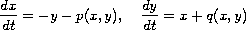where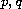are homogeneous polynomials of degree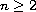. Associated with this system is an Abel differential equation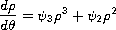in which the coefficients are trigonometric polynomials. We investigate two separate conditions which produce a constant first absolute invariant of this equation. One of these conditions leads to a new class of integrable, center conditions for the Poincare problem if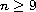is an odd integer. We also show that both classes of solutions produce polynomial solutions to the problem.

Submitted June 7, 2010. Published September 14, 2010.
Math Subject Classifications: 34A05, 34C25.
Key Words: Center-focus problem; Abel differential equation; constant invariant; symmetric centers.

Show me the PDF file (226 KB), TEX file, and other files for this article.Gary R. Nicklason Mathematics, Physics and Geology Cape Breton University Sydney, Nova Scotia, Canada B1P 6L2 email: gary_nicklason@capebretonu.ca